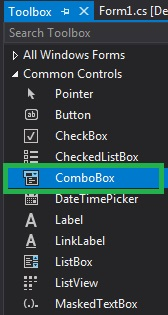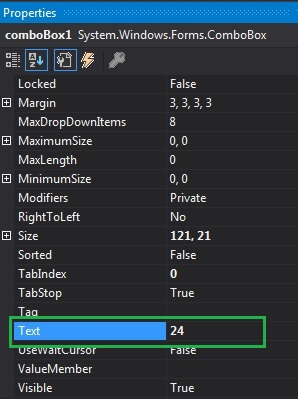Related Articles
How to set Text in ComboBox in C#?
• Last Updated : 30 Jun, 2019

In Windows Forms, ComboBox provides two different features in a single control, it means ComboBox works as both TextBox and ListBox. In ComboBox, only one item is displayed at a time and the rest of the items are present in the drop-down menu. You are allowed to add text in your ComboBox by using the Text Property. You can set this property using two different methods:

1. Design-Time: It is the easiest method to set the text in the ComboBox control using the following steps:

• Step 1: Create a windows form as shown in the below image:
Visual Studio -> File -> New -> Project -> WindowsFormApp• Step 2: Drag the ComboBox control from the ToolBox and drop it on the windows form. You are allowed to place a ComboBox control anywhere on the windows form according to your need.• Step 3: After drag and drop you will go to the properties of the ComboBox control to set the text in the ComboBox.Output:2. Run-Time: It is a little bit trickier than the above method. In this method, you can set the text in the ComboBox programmatically with the help of given syntax:

`public override string Text { get; set; }`

Here, the value of this property is of System.String type. Following steps are used to set the text in the ComboBox elements:

• Step 1: Create a combobox using the ComboBox() constructor is provided by the ComboBox class.
```// Creating ComboBox using ComboBox class
ComboBox mybox = new ComboBox();
```
• Step 2: After creating ComboBox, set the text in the ComboBox elements.
```// Set text in the combobox
mybox.Text = "Rohit";
```
• Step 3: And last add this combobox control to form using Add() method.
```// Add this ComboBox to form
```

Example:

 `using` `System; ` `using` `System.Collections.Generic; ` `using` `System.ComponentModel; ` `using` `System.Data; ` `using` `System.Drawing; ` `using` `System.Linq; ` `using` `System.Text; ` `using` `System.Threading.Tasks; ` `using` `System.Windows.Forms; ` ` `  `namespace` `WindowsFormsApp14 { ` ` `  `public` `partial` `class` `Form1 : Form { ` ` `  `    ``public` `Form1() ` `    ``{ ` `        ``InitializeComponent(); ` `    ``} ` ` `  `    ``private` `void` `Form1_Load(``object` `sender, EventArgs e) ` `    ``{ ` `        ``// Creating and setting the properties of label ` `        ``Label l = ``new` `Label(); ` `        ``l.Location = ``new` `Point(222, 80); ` `        ``l.Size = ``new` `Size(99, 18); ` `        ``l.Text = ``"Enter name"``; ` ` `  `        ``// Adding this label to the form ` `        ``this``.Controls.Add(l); ` ` `  `        ``// Creating and setting the properties of comboBox ` `        ``ComboBox mybox = ``new` `ComboBox(); ` `        ``mybox.Location = ``new` `Point(327, 77); ` `        ``mybox.Size = ``new` `Size(216, 26); ` `        ``mybox.MaxLength = 3; ` `        ``mybox.Text = ``"Rohit"``; ` ` `  `        ``// Adding this ComboBox to the form ` `        ``this``.Controls.Add(mybox); ` `    ``} ` `} ` `} `

Output:My Personal Notes arrow_drop_up
Recommended Articles
Page :